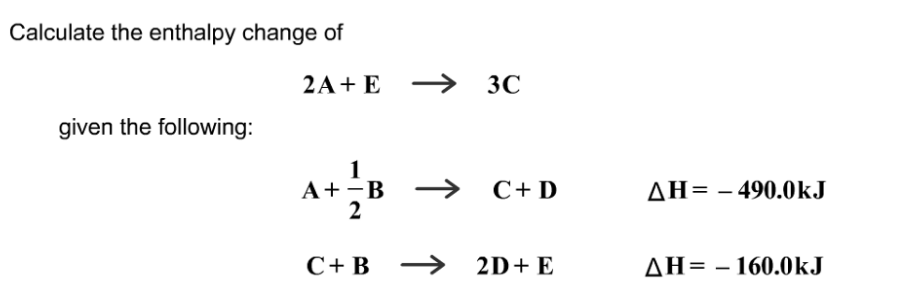# Problem: Calculate the enthalpy change of2A + E --&gt;3Cgiven the following:A + 1/2B --&gt; C + D Delta H = -490.0 kJC + B --&gt; 2D + E Delta H = -160.0 kJ

###### FREE Expert Solution
83% (380 ratings)###### Problem Details

Calculate the enthalpy change of
2A + E -->3C
given the following:
A + 1/2B --> C + D Delta H = -490.0 kJ
C + B --> 2D + E Delta H = -160.0 kJ